# Parameter-dependent integral

(diff) ← Older revision | Latest revision (diff) | Newer revision → (diff)

An integral of the type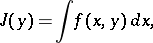in which the point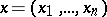ranges over the space(if the point ranges only over a certain domainin, the functionmay be assumed to vanish for), while the point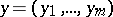, representing a set of parameters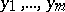, varies within some domainof the space.

The main concern of the theory of such integrals is to determine conditions for the continuity and differentiability ofwith respect to the parameters. Ifis interpreted as a Lebesgue integral, one obtains less restrictive conditions for its continuity and differentiability. The following propositions are valid.

1) If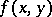is continuous inin the domainfor almost-alland if there exists an integrable functiononsuch that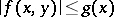for every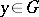and almost-all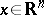, then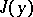is continuous in.

2) Let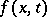be a function defined for,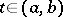. Assume that the derivativeexists for almost-alland everyand that is a continuous function ofon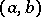for almost-all. Assume, moreover, that there exists an integrable functiononsuch that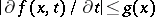for everyand almost-all. Finally, assume that for some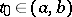the integralexists. Then the functionis differentiable with respect toon, and its derivativemay be evaluated by differentiating under the integral sign: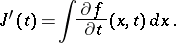These two propositions imply a series of simpler propositions about the continuity and differentiability of integrals with parameters, relating to the interpretation of the integral as a Riemann integral and to more specific cases (see ).

## Parameter-dependent improper integrals.

For the simplest improper integral of the first kind,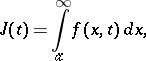(*)

one introduces the notion of uniform convergence with respect to the parameterin a closed interval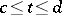. This integral is said to be uniformly convergent inon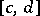if, for each, there exists an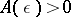such that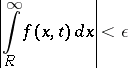for all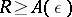.

The following propositions are valid.

a) Ifis continuous in a half-stripand if the integral (*) is uniformly convergent inon, thenis continuous in.

b) Ifand the derivative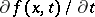are continuous in a half-strip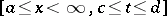, if the integral (*) is convergent for some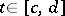and if the integral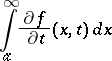is uniformly convergent inon, then the function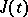is differentiable onand its derivative may be evaluated by the formula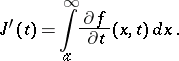Analogous propositions hold for improper integrals of the second kind.

How to Cite This Entry:
Parameter-dependent integral. Encyclopedia of Mathematics. URL: http://encyclopediaofmath.org/index.php?title=Parameter-dependent_integral&oldid=18531
This article was adapted from an original article by V.A. Il'in (originator), which appeared in Encyclopedia of Mathematics - ISBN 1402006098. See original article